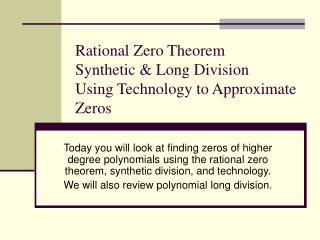DownloadDownload PresentationRational Zero Theorem Synthetic & Long Division Using Technology to Approximate Zeros

# Rational Zero Theorem Synthetic & Long Division Using Technology to Approximate Zeros

Télécharger la présentation## Rational Zero Theorem Synthetic & Long Division Using Technology to Approximate Zeros

- - - - - - - - - - - - - - - - - - - - - - - - - - - E N D - - - - - - - - - - - - - - - - - - - - - - - - - - -
##### Presentation Transcript

1. Rational Zero TheoremSynthetic & Long DivisionUsing Technology to Approximate Zeros Today you will look at finding zeros of higher degree polynomials using the rational zero theorem, synthetic division, and technology. We will also review polynomial long division.

2. Try Factor by Grouping!

3. Factor by grouping worked fine for our first example, but not for the second example so we need another way to find the roots for our second equation. We could just keep trying numbers until we find something that works OR We can use the Rational Zero Theorem to accomplish this more efficiently.

4. Getting to the Root of the (Polynomial) Matter You are often asked to find all the zeros (roots or x-intercepts) of polynomials. To do this in the most efficient way, use the rational zero test. • First, there are some general concepts. • When you FOIL a pair of quadratic binomials with leading coefficients of one, notice that for y = (x – r1)(x – r2) , the constant in the trinomial function is the product (r1 r2). • When we to do the multiplication for y = (x – r1)(x – r2)(x – r3), the constant in the polynomial function is the product (r1 r2 r3). • That means that all rational zeros must be in thefactor listfor the constant in a polynomial function when the leading coefficient is one.

5. Getting to the Root of the (Polynomial) Matter

6. Rational Zero Theorem If f(x) = anxn + . . . + a1x + a0 has integer coefficients, then every rational zero of f has the form: p factor of constant term a0 (c) --- = ----------------------------------------- q factor of leading coefficient an (a)

7. Find all possible rational zeros of the functions below using the Rational Zero Thm.

8. Let’s look at our example again: Let’s apply the Rational Zero Theorem to find all possible rational zeros and then we can use Synthetic Division to test those zeros.

9. Testing the possible rational zeros using Synthetic Division. Find the zeros of • Find all possible rational zeros using Rational Zero Theorem • Use synthetic division to test rational zeros • Factor or use quadratic formula (if you are left with a quadratic function) to find remaining zeros or use synthetic division again until you are left with a quadratic function.

10. More Examples to Try

11. Use Polynomial Long Division to Simplify

12. That means we must have Irrational Zeros. Because they are irrational, we need to use our graphing calculators to approximate the zeros. First, always graph the function. The number of times the function crosses the x-axis gives us the number of real zeros. What happens if we use synthetic division and none of the possible rational zeros work?

13. Zeros of Polynomial Functions Using a Graphing Calculator Approximate the real zeros of 1. Enter equation into Y1 2. Graph the equation 3. Go to CALC: zero 4. Move cursor to left of the zero – hit enter 5. Move cursor to right of zero – hit enter 6. Hit Enter one more time to see the zero. 7. The coordinates of the zero appear. Repeat the process to find all other real zeros.

14. Zeros of Polynomial Functions Using a Graphing Calculator Try this one: Approximate the real zeros of

15. Assignment A 1.9 Sect II & III A 1.10 (Book Reference: Section 2.4 pgs. 214 – 223) See yu tmrrw!